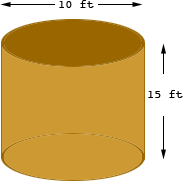Question: How many barrels of oil (42 gallons of oil in one oil tank) would there be in an oil tank that was 15 feet tall and 10 feet across? Thanks. Frances bookkeeper Frances,   I assume you mean a cylindrical tank.The volume V of a circular cylinder of radius r and height h is V =r2 h Your cylinder has radius 5 feet and height 15 feet so the volume is V =5 215 = 1178 cubic feet = 1178123 = 2 035 752 cubic inches. An American gallon is 231 cubic inches so your tank holds   2035752/231 = 8813 gallons. There are 42 gallons of oil in a barrel so your tank holds   8813/42 = 210 barrels. Penny